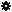﻿ Object Model: Geometry.GetRaycastIntersections

Geometry.GetRaycastIntersections

## Geometry.GetRaycastIntersectionsv6.0

### Description

Returns a PointLocatorData object containing the surface intersections found by raycasting from the specified input positions and rays. By default, input positions have to be defined in the object's local space reference.

In order to use this method you first need to specify siClosestSurfaceRaycastIntersection in the Method argument of Geometry.SetupPointLocatorQueries.

When you call this method an acceleration cache is automatically created. See Geometry.SetupPointLocatorQueries for more information.

Notice that the returned point locators can be evaluated on any Geometry instance having the same topology.

### C# Syntax

 ```PointLocatorData Geometry.GetRaycastIntersections( Object in_pPositions, Object in_pRays, siLineIntersectionType in_eLineType ); ```

### Scripting Syntax

 ```oReturn = Geometry.GetRaycastIntersections( Positions, Rays, LineType ); ```

### Parameters

Parameter Type Description
Positions Array An array of Doubles, either formatted as a 1D array of packed XYZ triplets, or as a 2D array of XYZ triplets.
Rays Array An array of Doubles, either formatted as a 1D array of packed XYZ triplets, or as a 2D array of XYZ triplets. Each one of these rays defines the vector used for the raycasting and whose length is important if you pass siSegmentIntersection for the LineType argument.
LineType siLineIntersectionType The type of line.

Default Value: siSemiLineIntersection

### Examples

#### JScript Example

 ```/* Example using GetRaycastIntersections to find the intersection with a ray and a mesh sphere */ NewScene(); var NullObj = Application.ActiveSceneRoot.AddNull(); Translate(NullObj, -2.0, 8.0, 5.0, siRelative, siView, siObj, siXYZ); var SphereObj = Application.ActiveSceneRoot.AddGeometry("Sphere", "MeshSurface"); var SphereGeom = SphereObj.ActivePrimitive.Geometry; var vbArr = new VBArray( NullObj.Kinematics.Global.Transform.GetTranslationValues2() ); var array = vbArr.toArray(); var vbArr2 = new VBArray( SphereObj.Kinematics.Global.Transform.GetTranslationValues2() ); var array2 = vbArr2.toArray(); array2 = array2 - array; array2 = array2 - array; array2 = array2 - array; var ClosestPointLocator = SphereGeom.GetRaycastIntersections( array, array2 ); var ClosestPosition = SphereGeom.EvaluatePositions(ClosestPointLocator).toArray(); var TriangleVertices = SphereGeom.GetTriangleVertexIndexArray(ClosestPointLocator).toArray(); var TriangleWeights = SphereGeom.GetTriangleWeightArray(ClosestPointLocator).toArray(); Application.LogMessage("The intersection of the mesh sphere and the ray starting where the Null is and ending at 0,0,0 is (" + ClosestPosition + ", " + ClosestPosition + ", " + ClosestPosition + ")"); Application.LogMessage("which corresponds to the triangle made of vertices (" + TriangleVertices + ", " + TriangleVertices + ", " + TriangleVertices + ")."); Application.LogMessage("The barycentric weight relatively to each triangle vertex is (" + TriangleWeights + ", " + TriangleWeights + ", " + TriangleWeights + ")."); //INFO : The intersection of the mesh sphere and the ray starting where the Null is and ending at 0,0,0 is (-0.7933665513992314, 3.1734662419917105, 1.9834164080087983) //INFO : which corresponds to the triangle made of vertices (14, 21, 22). //INFO : The barycentric weight relatively to each triangle vertex is (0.3966832756996155, 0.20538949966430664, 0.3979272246360779). ```